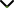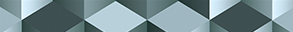# Inhomogeneous Nonlinear Partial Differential Problems: Existence and Non-Existence of Solutions

Publishing date
01 Aug 2021
Status
Closed
19 Mar 2021

Guest Editors

1King Saud University, Riyadh, Saudi Arabia

2University of Palermo, Palermo, Italy

4Yulin Normal University, Yulin, China

This issue is now closed for submissions.
More articles will be published in the near future.

# Inhomogeneous Nonlinear Partial Differential Problems: Existence and Non-Existence of Solutions

This issue is now closed for submissions.
More articles will be published in the near future.

## DescriptionIt is well known that many physical systems respond to the breaking of regularities, which is often useful to determine the mathematical form of the equations dictating the behaviour of special phenomena. For example, the solvability of existence and stability problems involving nonlinear damped wave equations on symmetric domains, and double phase (p,q)-Laplacian equations in Lebesgue and Sobolev spaces, both isotropic and anisotropic, play a crucial role in particle systems, acoustics, and elasticity theory.

We know from the literature that the solvability of such equations is strongly related to the critical and asymptotic behaviour of solutions. It is known that Schrödinger type equations arise naturally in the study of dispersive phenomena, as in the case of wave equations, and are useful tools to provide precise information on the existence and non-existence of positive solutions. On the other hand, the existing regularity theory for isotropic double phase equations in Lebesgue and Sobolev spaces with constant exponents does not cover anisotropic double phase equations in Lebesgue and Sobolev spaces with variable exponents.

The aim of this Special Issue is to study the qualitative behaviour of different inhomogeneous nonlinear partial differential problems. We look to collect results establishing both the existence and non-existence of "global weak solutions" with suitable regularity properties, by focusing on control tools, namely, critical exponent values and critical parameters in the reaction term of the equations, leading to bifurcation-type theorems. Both original research and review articles are welcome.

Potential topics include but are not limited to the following:

• Variational methods for Schrödinger type equations and (p,q)-Laplacian equations
• Mitidieri–Pohozaev capacity method for finite in time blow-up of solutions to nonlinear equations
• Fractional in time evolution equations
• Critical behaviour for nonlinear evolution equations
• Lifespan of solutions
• Maz'ya isocapacitary inequalities for double phase equations in Orlicz-Sobolev spaces
• Topological methods for nonlinear (p,q)-Laplacian type equations
• Extension of the known theories to the fractional versions of Schrödinger type equations
• Extension of the known theories to the fractional versions of (p,q)-Laplacian equations
• Variational inequalities governed by fractional Laplacian type operatorsJournal metrics
See full report
Acceptance rate33%
Submission to final decision52 days
Acceptance to publication26 days
CiteScore2.400
Journal Citation Indicator1.150
Impact Factor1.281Author guidelinesEditorial boardDatabases and indexing

Article of the Year Award: Outstanding research contributions of 2021, as selected by our Chief Editors. Read the winning articles.